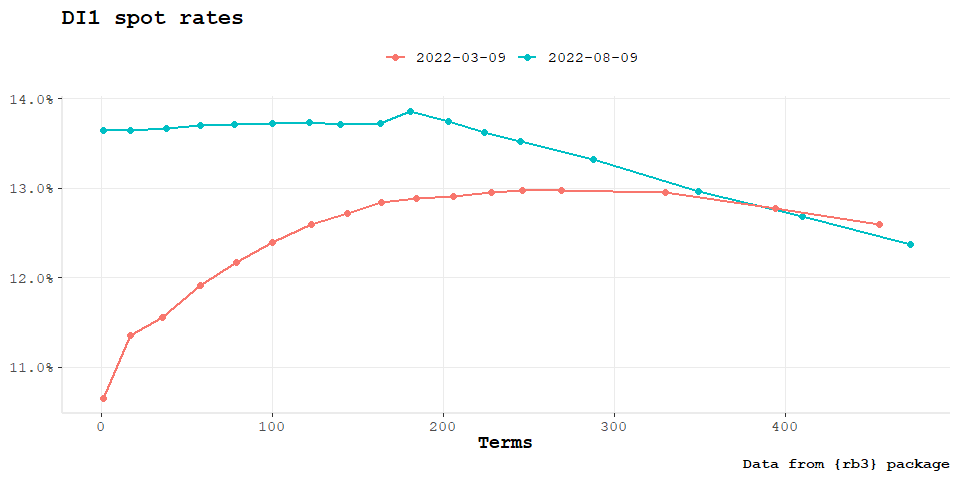# Plotting Spot Rate Curves

Visualization of yield curves is fairly relevant and the package fixedincome brings the usual plot function to create plots with the SpotRateCurve objects and also a ggplot2 set of methods that helps with the visualization of the term structures using all ggplot2 features.

Before the start let’s get the necessary packages loaded.

library(rb3)
library(dplyr)
library(fixedincome)
library(ggplot2)

As the second step, the yield curve must be created and this is done with data from {rb3} package.

refdate <- as.Date("2022-08-09")
yc_ <- yc_get(refdate)
fut_ <- futures_get(refdate)
yc_ss <- yc_superset(yc_, fut_)
yc <- bind_rows(
yc_ss |> slice(1),
yc_ss |> filter(!is.na(symbol))
) |>
filter(!duplicated(biz_days))

curve <- spotratecurve(
yc$r_252, yc$biz_days, "discrete", "business/252", "Brazil/ANBIMA",
refdate = refdate
)

## base::plot

plot(curve)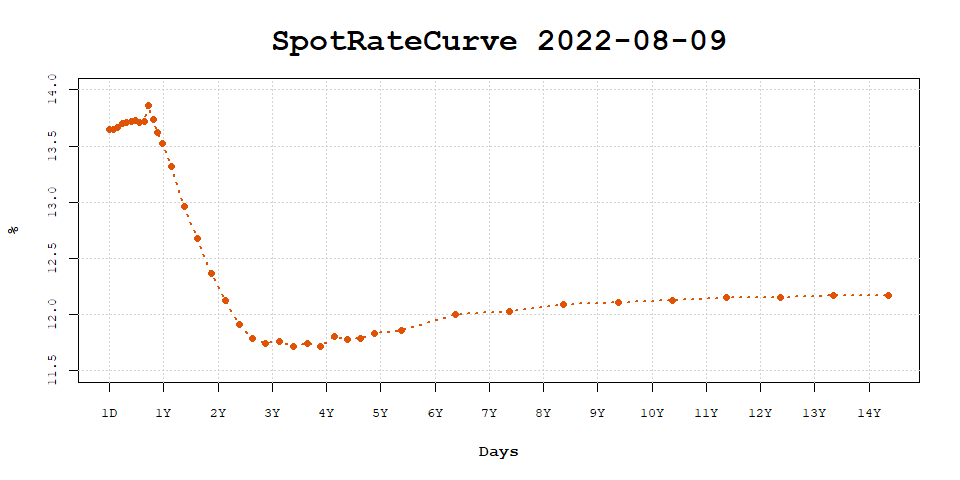plot(curve, show_forward = TRUE)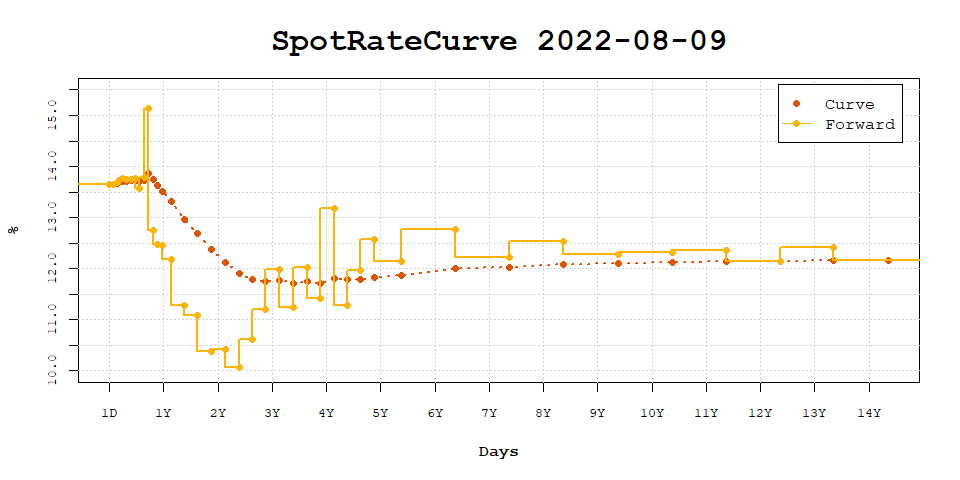curve |>
fixedincome::first("3 years") |>
plot(curve, show_forward = TRUE)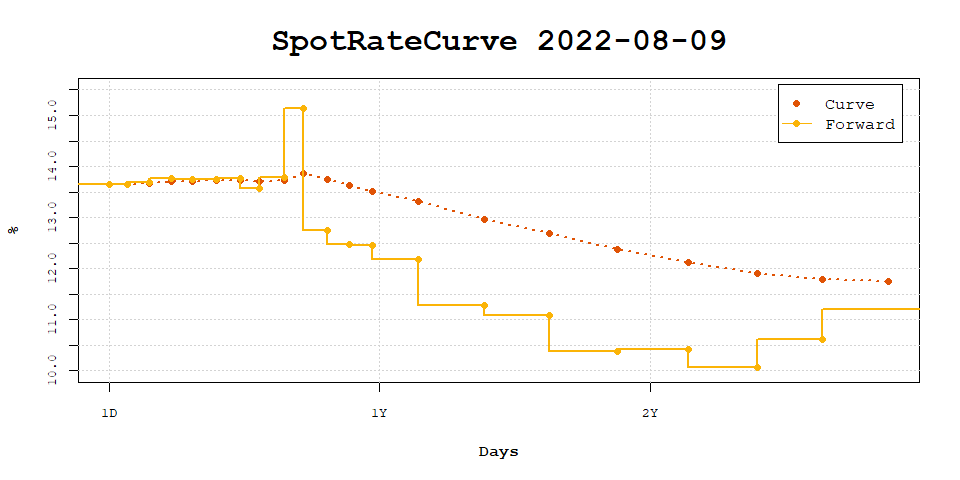curve_2y <- curve |> fixedincome::first("2 years")
interpolation(curve_2y) <- interp_naturalspline()
plot(curve_2y, use_interpolation = TRUE)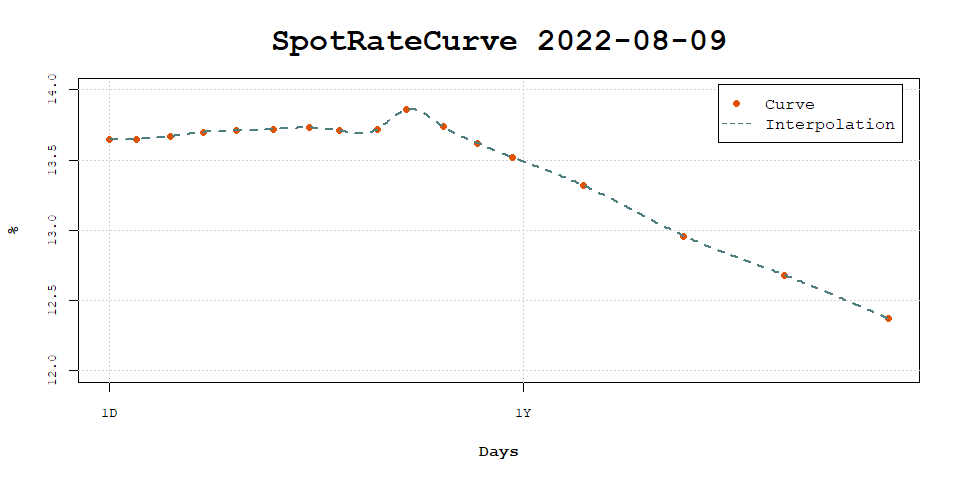plot(curve_2y, use_interpolation = TRUE, show_forward = TRUE)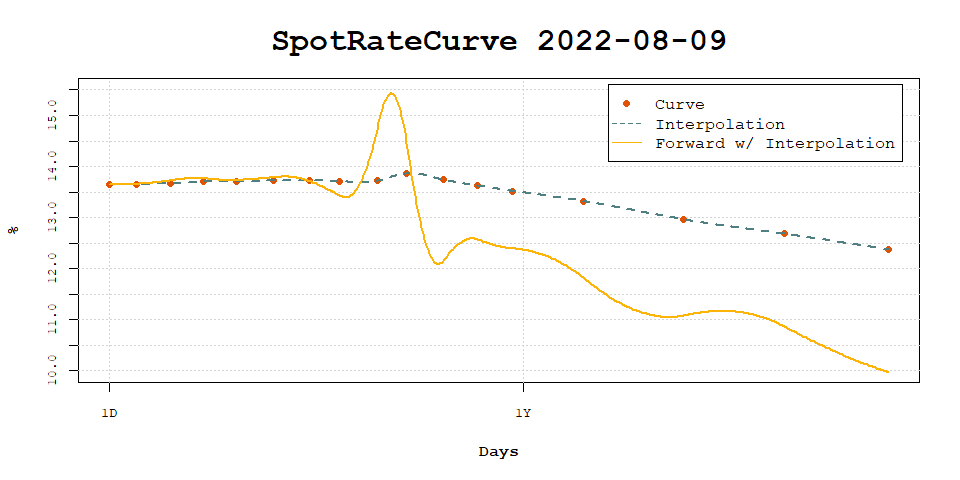plot(curve_2y, use_interpolation = TRUE, show_forward = TRUE, legend_location = "bottomleft")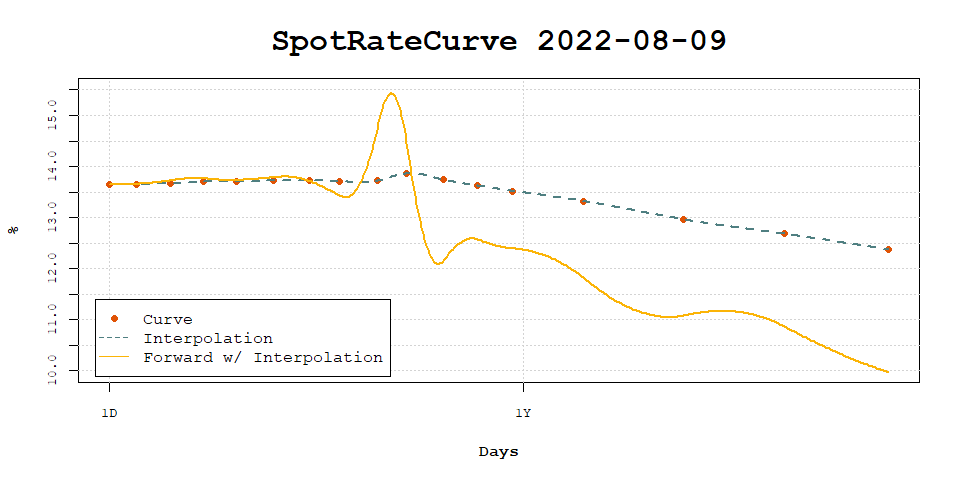## ggplot2

ggspotratecurveplot(curve,
title = "DI1 spot rates", subtitle = format(refdate), caption = "Data from {rb3} package"
)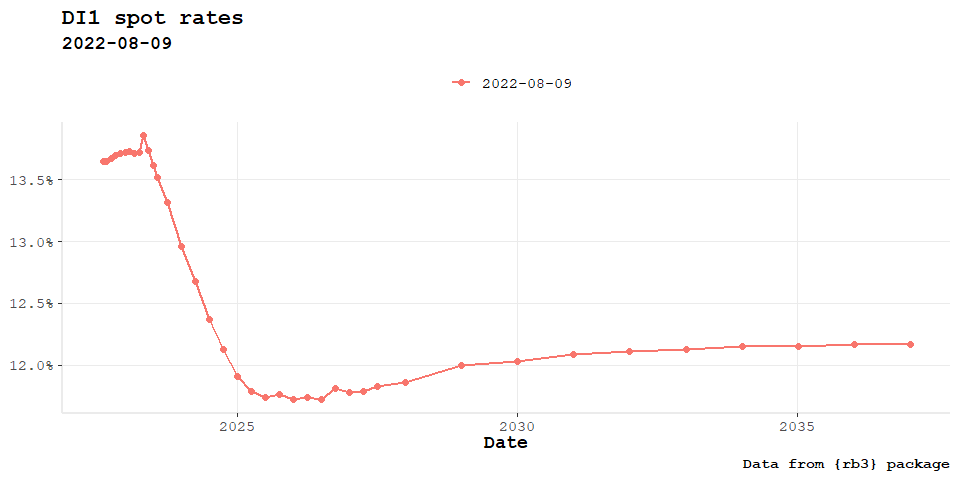ggspotratecurveplot(curve,
title = "DI1 spot rates", subtitle = format(refdate), caption = "Data from {rb3} package",
curve.x.axis = "terms"
)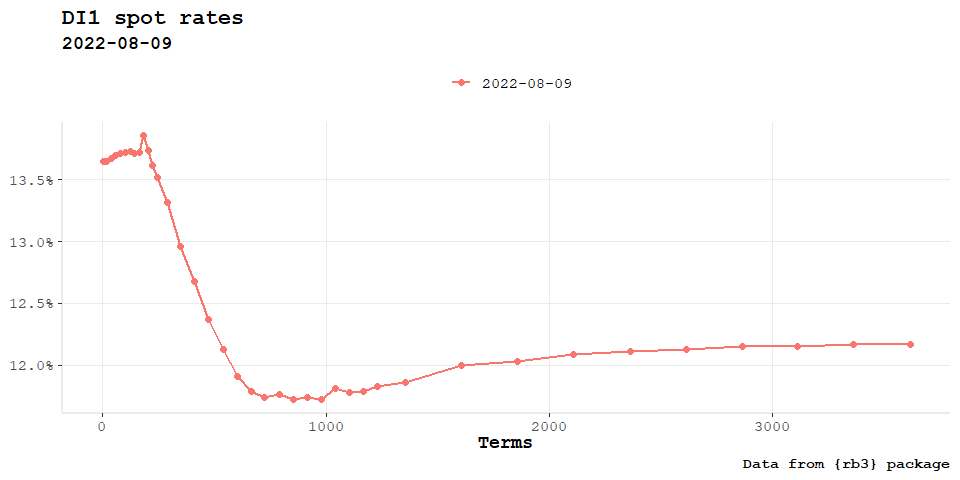ggspotratecurveplot(curve,
title = "DI1 spot rates", subtitle = format(refdate), caption = "Data from {rb3} package"
) +
autolayer(forwardrate(curve), size = 1)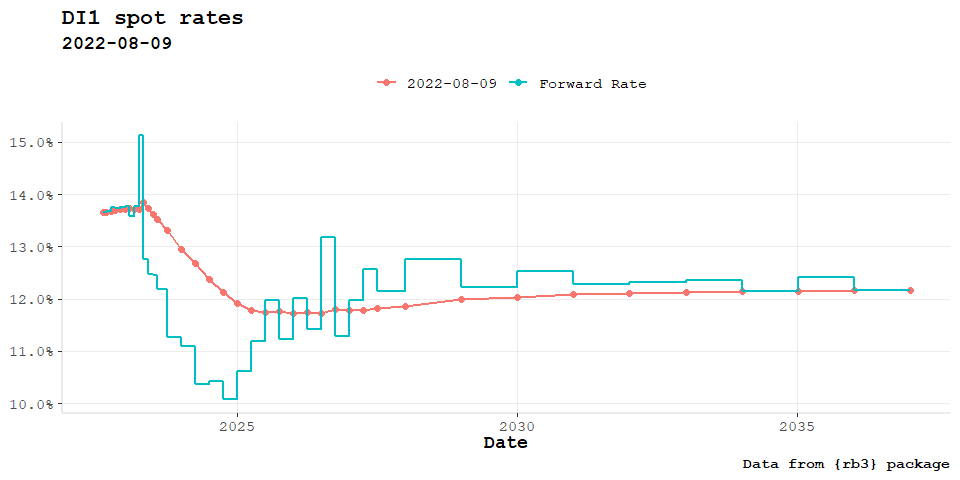ggspotratecurveplot(curve_2y,
title = "DI1 spot rates", subtitle = format(refdate), caption = "Data from {rb3} package",
curve.interpolation = TRUE
)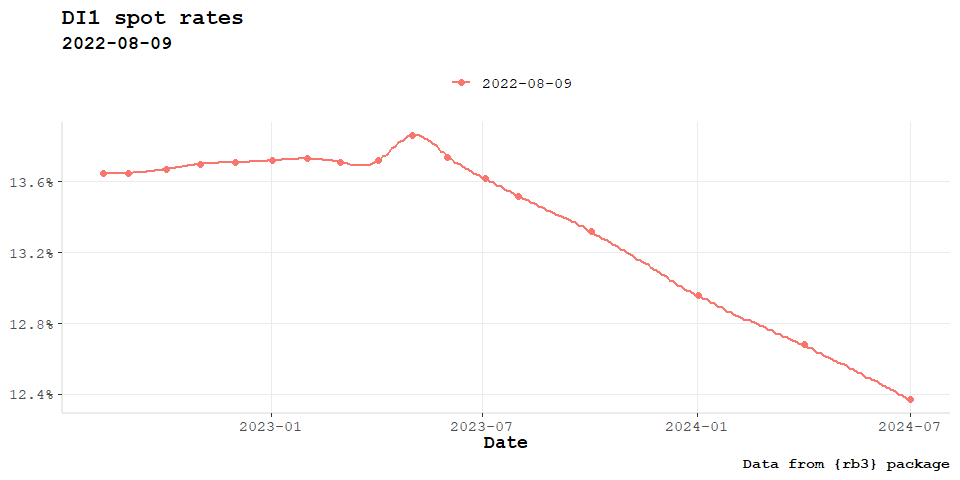autoplot(curve_2y, curve.geom = "point") +
autolayer(curve_2y, curve.geom = "line", curve.name = "Interpolation", curve.interpolation = TRUE)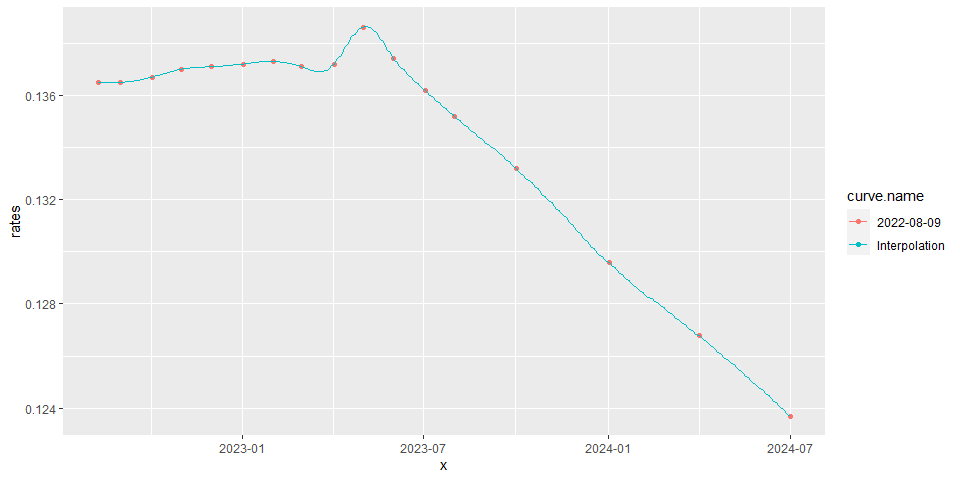terms_ <- do.call(seq, as.list(range(curve_2y@terms)))
curve_2y_interp <- curve_2y[[terms_]]
autoplot(curve_2y, curve.geom = "point") +
autolayer(curve_2y_interp, curve.geom = "line") +
autolayer(forwardrate(curve_2y_interp),
curve.geom = "line", curve.name = "Forward Rate"
)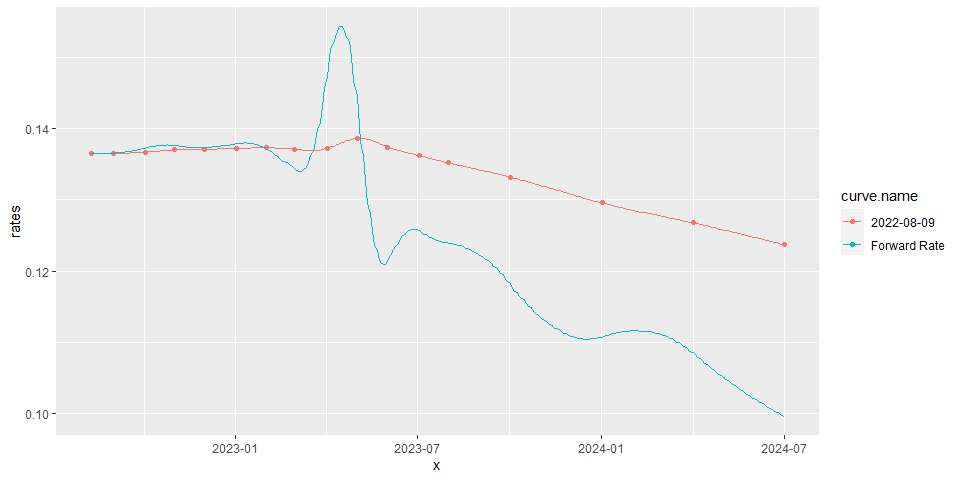### Plotting Multiple Curves

refdate2 <- as.Date("2022-03-09")
yc_ <- yc_get(refdate2)
fut_ <- futures_get(refdate2)
yc_ss <- yc_superset(yc_, fut_)
yc <- bind_rows(
yc_ss |> slice(1),
yc_ss |> filter(!is.na(symbol))
) |>
filter(!duplicated(biz_days))

curve2 <- spotratecurve(
yc$r_252, yc$biz_days, "discrete", "business/252", "Brazil/ANBIMA",
refdate = refdate2
)
ggspotratecurveplot(curve,
title = "DI1 spot rates", caption = "Data from {rb3} package",
curve.x.axis = "terms"
) +
autolayer(curve2, curve.x.axis = "terms", size = 1) +
autolayer(curve2, curve.geom = "point", curve.x.axis = "terms", size = 2)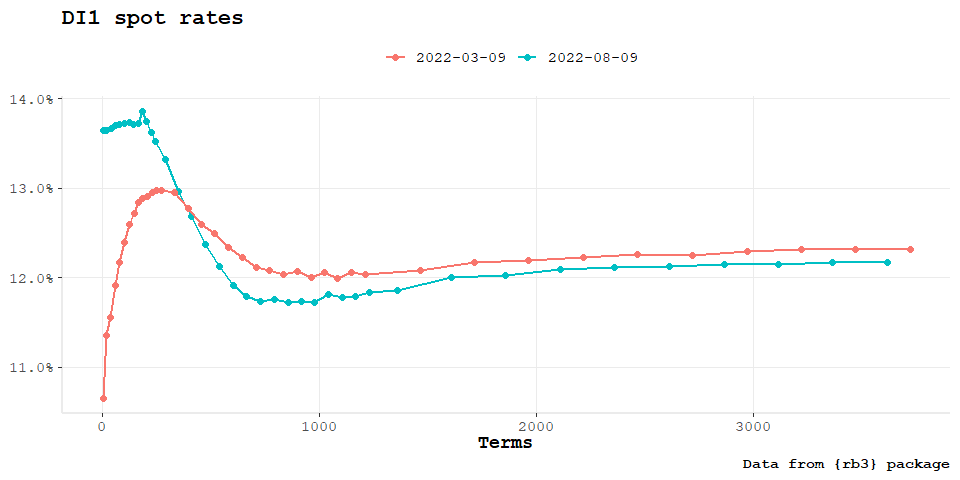curve2_2y <- curve2 |> fixedincome::first("2 years")
ggspotratecurveplot(curve_2y,
title = "DI1 spot rates", caption = "Data from {rb3} package",
curve.x.axis = "terms"
) +
autolayer(curve2_2y, curve.x.axis = "terms", size = 1) +
autolayer(curve2_2y, curve.geom = "point", curve.x.axis = "terms", size = 2)